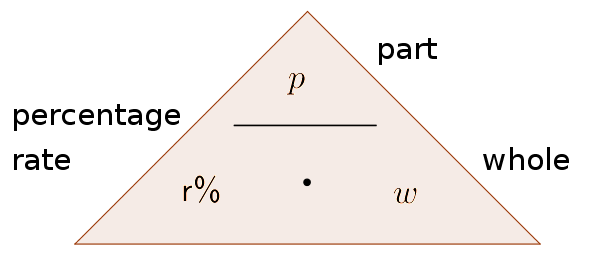Math Percentage calculation Percentage calculation

# Percentage calculation

If two or three sizes are known, you can use this triangle as a reminder in order to calculate the third size.The wanted size has to be covered with a finger. This is how you get the formula.

## Original value

The original value $w$ is the whole thing which can be compared to something. The formula is:

$w=\frac{p}{r\%}$

10% of the total number, which is unknown, is 50€.

It is given: $p=50€$ und $r\%=10\%$

$w=\frac{p}{r\%}$
$w=50€:10\%$ $=50€:\frac{10}{100}$ $=50€\cdot\frac{100}{10}$ $=500€$

## Percentage value

The percentage value $p$ (new value) is part of the whole and it shows how much it shares of the original value.

$p=r\%\cdot w$

### Example

Find 10% of 500€

It is given: $w=500€$ und $r\%=10\%$

$p=r\%\cdot w$
$p=10\%\cdot 500€$ $=\frac{10}{100}\cdot 500€$ $=50€$

## Percentage rate

The percentage rate $r\%$ shows how large the amount, of the percentage, is of the original value.

$r\%=\frac{p}{w}$

### Example

What percent is 50€ of 500€?

It is given: $w=500€$ und $p=50€$

$r\%=\frac{p}{w}$
$r\%=\frac{50€}{500€}$ $=\frac{10}{100}$ $=10\%$

!

### Attention

$r\%=\frac{r}{100}$

In order to calculate the percent rate it is important to divide it by 100.
Example: $7\%=\frac7{100}=0.07$ and not 7.